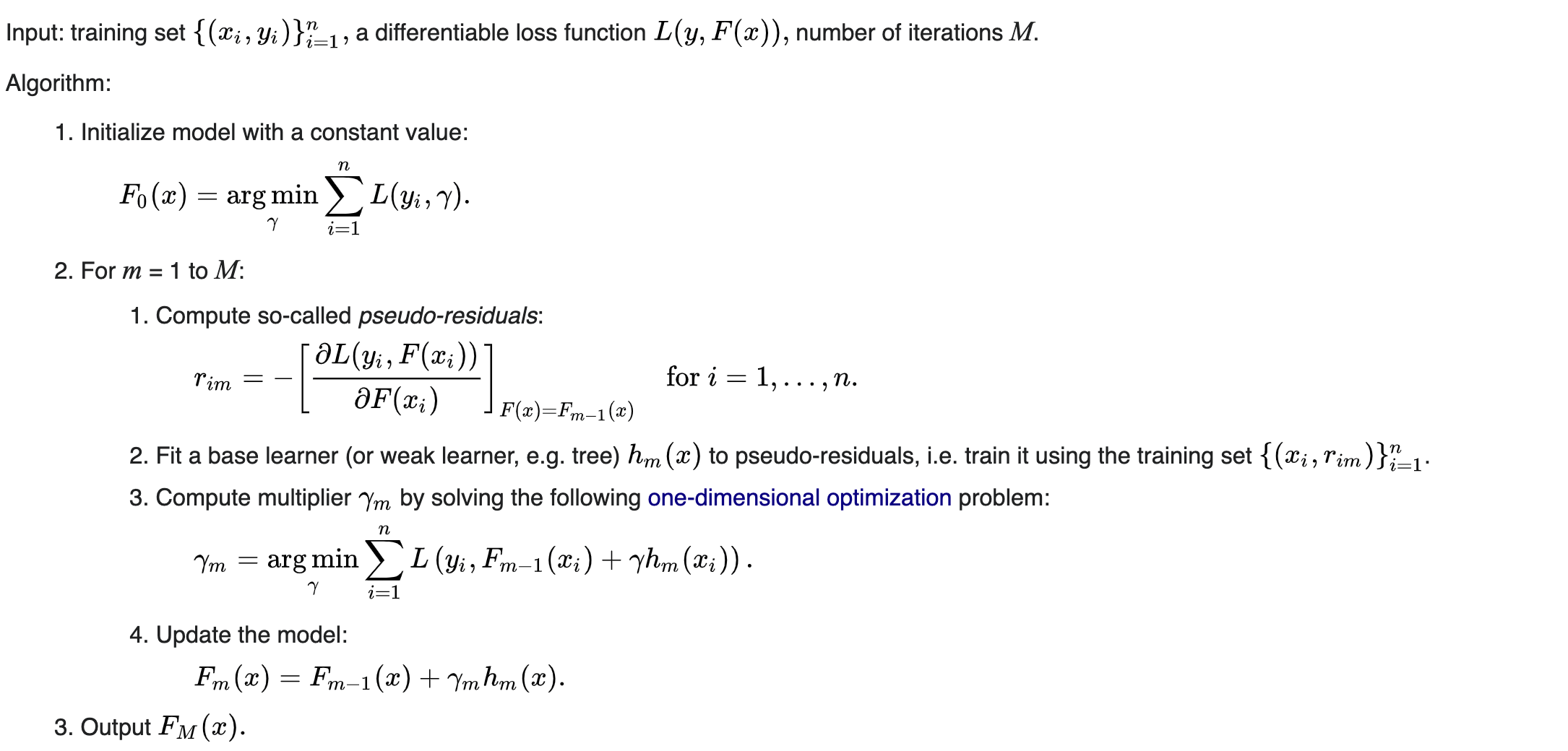### Bounty: 50Above is the pseudocode for gradient boosting. In Step 2.3, we’re computing a multiplier (or step length) $$gamma_m$$. Suppose the loss function $$L(y_i, hat{y}_i) = frac{1}{2}(y_i – hat{y}_i)^2$$. Then to find $$gamma_m$$, we would have

begin{align} gamma_m &= text{arg min}gamma frac{1}{2}sum{i = 1}^n (y_i – F_{m-1}(x_i) – gamma h_m(x_i))^2 end{align}

Taking the derivative wrt $$gamma$$, we have

begin{align} frac{partial}{partial gamma} frac{1}{2}sum_{i = 1}^n (y_i – F_{m-1}(x_i) – gamma h_m(x_i))^2 &= -sum_{i=1}^n h_m(x_i)(y_i – F_{m-1}(x_i) – gamma h_m(x_i))\ &= -sum_{i=1}^n h_m(x_i)(y_i – F_{m-1}(x_i) + gamma sum_{i=1}^n h_m^2(x_i)\ & overset{set}{=}0\ Rightarrow gamma_m &= frac{sum_{i=1}^n h_m(x_i)(y_i – F_{m-1}(x_i))}{sum_{i=1}^n h_m(x_i)^2} end{align}

Is this correct? If so, what’s the intuition behind this step length $$gamma$$? In my own implementation of this algorithm, I’ve been computing $$gamma_m = frac{sum_{i=1}^n h_m(x_i)(y_i – F_{m-1}(x_i))}{sum_{i=1}^n h_m(x_i)^2}$$ and the values of $$gamma_m$$ are all very close to 1. What does that suggest about my algorithm?

Get this bounty!!!

This site uses Akismet to reduce spam. Learn how your comment data is processed.